# LoRa Location Tracker Part 6 (Calculation)

Starting from where I stopped before. Now I can move on to the final part of my project – getting the actual location of the target to make sure the elderly is inside the home and get the notification if the elderly leaves the home.

First, let me have a quick recall about the setup of my whole system which I mentioned in Part 1. In total I have 5 receivers, 4 of them are setup in each corner of the elderly home, and one setup in the exit. Each receiver is using an “Adafruit RFM96W LoRa RF Transceiver Breakout Board” connect to an “Arduino nano 33 IOT” sending the RSSI value back to the Raspberry Pi through Wi-Fi and store in the database.

To evaluate the performance of this system, we build up the system inside a venue (see Fig. 1). The dimension of the venue is 3 m × 6 m. There are two users (i.e., T1 and T2) and five receivers (i.e., R1 to R5) in the venue. The receivers are connected to the server through Wi-fi. Note that R5 is the “Exit Receiver”. It is used to estimate the distance between the user (transmitter) and the venue exit. The coordinates of R1 to R5 are (0, 0), (0, 6), (3, 6), (3, 0) and (1.5, 0) respectively (unit in m).

As I mentioned in part 2, RSSI values are converted to distances in the Raspberry Pi via the RSSI-Distance conversion equation.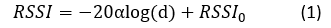By using Eq. (1), we can get the distance between a user (transmitter) i and a receiver j (i.e., dij) from the following equation: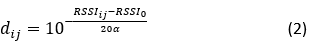where RSSIij is the measured RSSI value of the signal transmitted from the user i to the receiver j, RSSI0 is the reference RSSI value over one meter, and α is the path loss index. Note that all these three values are obtained by measurement.

For each receiver, they are following the below equation to get the transmitter coordinate: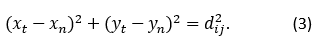Take T1 as an example. We measure the RSSI values from the four receivers R1, R2, R3, and R4, R5.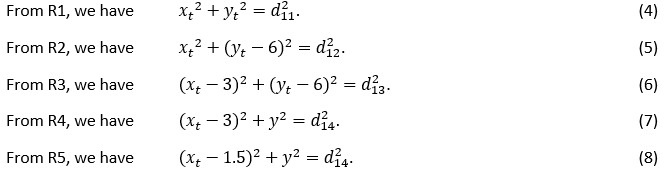To simplify the calculation, we consider ten sets of receivers (). Set A is (R1, R2, R3), Set B is (R2, R3, R4), Set C is (R1, R3, R4), Set D is (R1, R2, R4), Set E is (R3, R2, R5), Set F is (R1, R2, R5), Set G is (R3, R4, R5), Set H is (R1, R3, R5), Set I is (R2, R4, R5), Set J is (R1, R4, R5). But in our setting, since Set J receivers are placed in parallel, we cannot use Set J to find the transmitter's position. Therefore, in total, we have nine sets of receivers. For each set of equations, we can calculate as a matrix, take Set A (R1, R2, R3) as an example, i.e.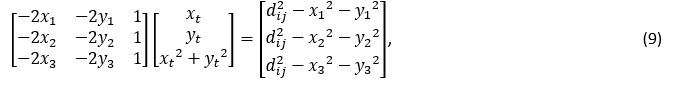Thought equation we can get Set A (R1, R2, R3) xy-coordinate (), then the estimated coordinate of the user is the average of the solutions of nine equations like above, i.e.,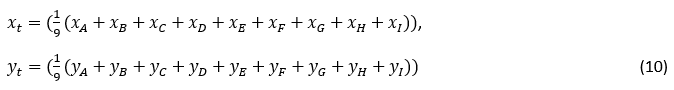Note that R5 is also used to determine whether a user is inside or outside the venue. It is close to the exit and thus the measured RSSI value of signals received by R5 can help to precisely confirm whether the above issue happens or not.

In the above method, we can through the x-coordinate and y-coordinate to check whether the target escapes the elderly home. Also, as I set up a receiver (R5) in the exit, therefore for the use of R5 is only measuring the distance between the target and the elderly home if the target leaves the elderly home.

Using the above methods, in the table below, we can see that the system can correctly identify transmitters inside and outside the venue at this stage. If we determine the actual coordinates of the transmitter inside the venue and without walls blocking the signal, the accuracy is around +-1m.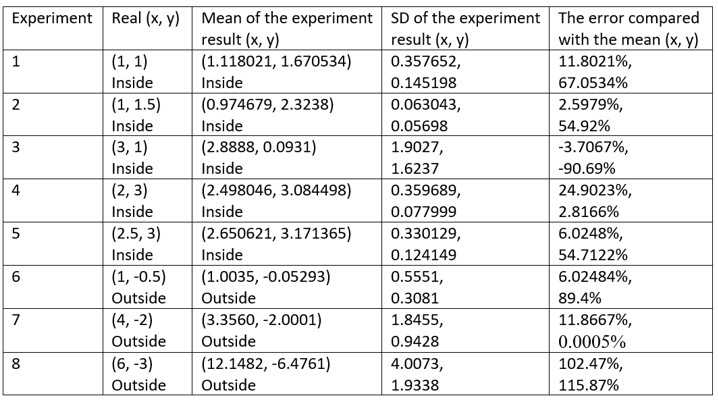However, when we determine the actual coordinates of the transmitter outside the venue and with the walls obstructing the signal, the accuracy will decline obviously, and the error depends on the materials and the thickness of the wall.

Finally, thank you for reading this series of articles about LoRa Location Trackers, I hope you enjoy it.

Here's a demo of the whole project, hope it helps you become more familiar with what I'm doing in this project! thank you very much!

JulianWong has not written a bio yet…